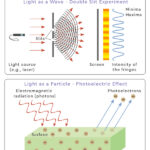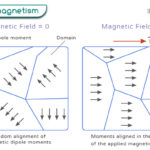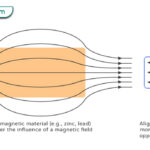Home / Physics / Newton’s Law of Cooling

# Newton’s Law of Cooling

Newton’s law of cooling is an empirical law to model the temperature of an object based on radiative cooling. It states that the object’s temperature changes at a rate proportional to the temperature difference between the object and its surroundings. This law is a reasonably accurate approximation to some extent.

The law is named after British mathematician Isaac Newton, who published it in 1701. Newton did not initially state his law in its current form. It was because of the confusion arising from the concept of heat and temperature, which did not exist during his time.

## Mathematical Form of the Law

Let T denote the object’s temperature and To denote the temperature of its surroundings, also known as the ambient temperature. Suppose t represents the time. According to Newton’s law of cooling,

$\frac{dT}{dt} = -k(T – T_o)$

where k is a positive constant known as the cooling constant. The negative sign implies that the object’s temperature is decreasing with time.

Therefore, an object’s cooling rate depends on the following:

• the temperature difference between it and its surroundings
• the cooling constant

From the above equation, we can make the following conclusions:

• If the object is much hotter than its surroundings, T – To is large and has a high cooling rate. The object cools rapidly.
• If the object is slightly hotter than its surroundings, T – To is small, and the cooling rate is low. The object cools slowly.

It means a cup of hot coffee cools more rapidly when kept in the fridge than outside.

## Newton’s Law of Cooling Formula

The mathematical form of Newton’s law of cooling is a differential equation of first order. It can be solved by rearranging the equation and integrating both sides.

$\frac{dT}{dt} = – k (T – T_o) \\ => \frac{dT}{T – T_o} = – k dt \\ => {\int_{T_i}}^{T_f} \frac{dT}{T – T_o} = – k {\int_0}^{t} dt \\ => \ln \frac{T_f – T_o}{T_i – T_o} = -kt \\ => T_f – T_o = (T_i – T_o) e^{-kt} \\ => T_f = T_o + (T_i – T_o) e^{-kt}$

Ti is the object’s initial temperature, and Tf is its final temperature.

When t , Tf = To. The object attains the temperature of its surroundings eventually.

## Limitations

Newton’s law of cooling is limited to the following conditions.

• The temperature difference between the object and its surroundings must be low.
• Only heat lost by radiation is considered.
• The temperature of the surrounding must remain constant during the cooling process.

## Example Problems

Problem 1. Water is heated to 70 oC and allowed to cool for 5 minutes. What would be its temperature if k = 0.051 min-1 and the surrounding temperature is 25 oC?

Solution.

Given Ti = 70 oC, To = 25 oC, t = 5 mins, k = 0.051 min-1

We use the following equation.

Tf = To + (Ti – To) e-kt

=> Tf = 25 + (70 – 25) exp (-0.051 x 5) = 59.9 oC

Problem 2. A body with a temperature of 50 oC is kept in an environment of 25 oC. If the body’s temperature falls to 45 oC in 10 minutes, find out how much excess time it will take for the body to attain a temperature of 40 oC.

Solution.

Given Ti = 50 oC, Tf = 45 oC, To = 25 oC, t = 10 mins

We use the following equation to find k.

Tf = To + (Ti – To) e-kt

=> k = (1/t) ln[(Ti – To)/(Tf – To)]

=> k = (1/10 min) ln[(50 – 25)/(45 – 25)] = 0.051 min-1

For the second interval,

Ti = 45 oC, Tf = 40 oC, k = 0.051 min-1

t = (1/0.051 min-1) ln[(45 – 25)/(40 – 25)] = 5.64 min

Problem 3. A body cools from 75 °C to 55 °C in 5 minutes. The temperature of its surroundings is 25 °C. What will be its temperature after the next 5 minutes?

Solution.

Given Ti = 75 oC, Tf = 55 oC, To = 25 oC, t = 5 mins

We use the following equation.

Tf = To + (Ti – To) e-kt

=> k = (1/t) ln[(Ti – To)/(Tf – To)]

=> k = (1/5 min) ln[(75 – 25)/(55 – 25)] = 0.102 min-1

For the second interval,

Ti = 55 oC, t = 5 mins, k = 0.102 min-1

The final temperature is

Tf = To + (Ti – To) e-kt

=> Tf = 25 + (55 – 25) exp(-0.102 x 5) = 43 oC

Article was last reviewed on Friday, July 28, 2023

### Related articlesWave-Particle DualityPhotoelectric EffectFerromagnetismDiamagnetism